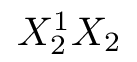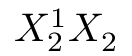Frequently Asked Question List for TeX

# Even subscript height

Other things being equal, TeX will aim to position subscripts and superscripts in places that “look good”. Unfortunately, it only does this for the sub- and superscripts of each atom at a time, so if you have

``````\$ X^{1}_{2} X_{2} \$
``````

the second subscript will appear higher, since the first has moved down to avoid the superscript; the effect can be noticeably distracting:You can avoid the problem, for a single instance, by

``````\$ X^{1}_{2} X^{}_{2} \$
``````

here, the dummy superscript has the requisite “pushing down” effect:While this technique does what is necessary, it is tedious and potentially error-prone. So, for more than one or two equations in a document, the LaTeX user is advised to use the `subdepth` package, which forces the lower position for all subscripts.

FAQ ID: Q-subheight
Tags: math